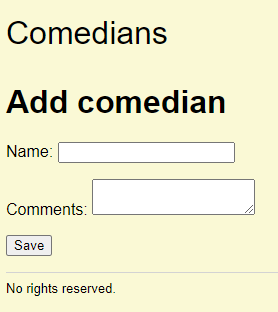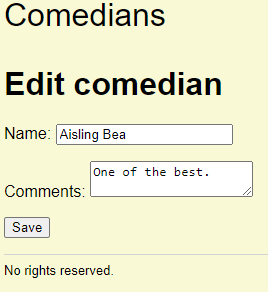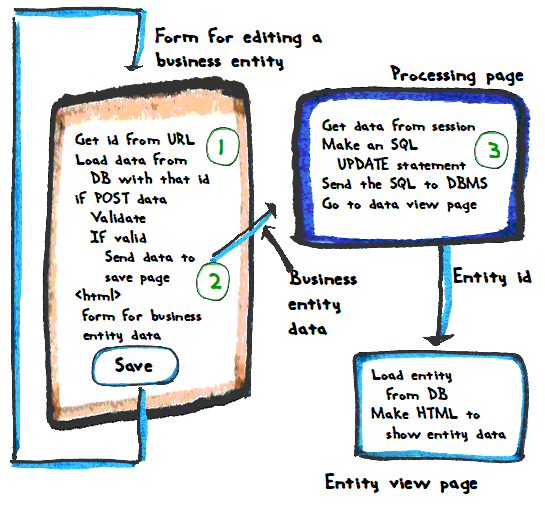... and the edit form...... are almost the same. Can we combine their code? Have one page to do both?Ethan

Why do that?

Principle

Reuse your code across programs, using functions and page components.

Good question, Ethan. The same code will work add and edit, or most of it will, anyway. If we change things, like add a new field to comedians, we only have to change one program, not two.

### Differences

Here's the edit workflow again.The differences from the add page are marked.

The edit page loads existing data from the DB (1), before showing the form. The add form doesn't do that.

The edit page sends the comedian's id to the processing page (2). The add form doesn't.

They use different SQL (3).

There are other minor things, like page title, but that's mostly it.

Our goal is to have one page, with one URL, handle the form for add and edit.

### How to tell edit from add?

We need a way on our form program to tell edit from add. How?Well, edit has an id, like, which comedian you're editing. When you add a new comedian, there isn't an id. Could we use that?

Hmm, maybe. How will we know whether the form has an id or not? There will be just one URL for both operations, remember.Georgina

Oo! Check the URL! Edit has `?=comedian_id=something` on the end. Add does not.

Right! If there's an id there, it's an edit. If not, it's an add.

### Code

We're going to look at code for the form, but before we do, notice that three variables are central:

• `\$comedianId`
• `\$comedianName`
• `\$comedianComments`

Each one is a field of the comedian entity. Let' call them field variables (FVs).

Here's how they change during the program.

• The field variables are set to MT.
• If it's an edit:
•   Replace the current values of the field variables with values from the DB.
• If there's POST data:
•   Replace the current values of the field variables with values from the POST.
•   If the field variables contain good data:
•     Put the field variables into the session.
• Show widgets, with values from the field variables.

OK, so what happens if the user (Alice) is starting to add a new record?Hi! I'm Alice!

• The field variables are set to MT. Do this, FVs MT
• If it's an edit: No, skip it, leave FVs MT
•   Replace the current values of the field variables with values from the DB.
• If there's POST data: No, skip it, leave FVs MT
•   Replace the current values of the field variables with values from the POST.
•   If the field variables contain good data:
•     Put the field variables into the session.
• Show widgets, with values from the field variables. Show MT widgets

That's what we want. MT widgets.

Now, suppose Alice is adding a new entity, types some invalid data, and hits the submit button. Here' what happens next.

• The field variables are set to MT. Do this, FVs MT
• If it's an edit: No, skip it, leave FVs MT
•   Replace the current values of the field variables with values from the DB.
• If there's POST data: There is
•   Replace the current values of the field variables with values from the POST. Do it
•   If the field variables contain good data: Nope
•     Put the field variables into the session.
• Show widgets, with values from the field variables. Show widgets with values from the POST

That's what we want. Alice sees the values to she typed.

Alice fixes the errors, and submits again:

• The field variables are set to MT. Do this, FVs MT
• If it's an edit: No, skip it, leave FVs MT
•   Replace the current values of the field variables with values from the DB.
• If there's POST data: There is
•   Replace the current values of the field variables with values from the POST. Do it
•   If the field variables contain good data: Yes
•     Put the field variables into the session. Do it
• Show widgets, with values from the field variables.

That's what we want. The processing page will get the values that were in the POST, which we know are valid.

OK, let's go to edit. Alice clicks a link to edit an existing record.

• The field variables are set to MT. Do this, FVs MT
• If it's an edit: Aye
•   Replace the current values of the field variables with values from the DB. Do this
• If there's POST data: Nope
•   Replace the current values of the field variables with values from the POST.
•   If the field variables contain good data:
•     Put the field variables into the session.
• Show widgets, with values from the field variables. Show widgets with data from the DB

That's what we want. The form shows the current data for the entity, from the DB.

Alice changes one of the fields, but makes an error.

• The field variables are set to MT. Do this, FVs MT
• If it's an edit: Aye
•   Replace the current values of the field variables with values from the DB. Do this
• If there's POST data: Aye
•   Replace the current values of the field variables with values from the POST. Do it
•   If the field variables contain good data: Nope
•     Put the field variables into the session.
• Show widgets, with values from the field variables. Show widgets with data from the POST

That's what we want.

Alice fixes everything.

• The field variables are set to MT. Do this, FVs MT
• If it's an edit: Aye
•   Replace the current values of the field variables with values from the DB. Do this
• If there's POST data: Aye
•   Replace the current values of the field variables with values from the POST. Do it
•   If the field variables contain good data: Indeed
•     Put the field variables into the session. Do it
• Show widgets, with values from the field variables. Show widgets with data from the POST

That's what we want. W00t!

Here's the code:

1. <?php
2. /**
5.  */
6. session_start();
7. require_once 'library/useful-stuff.php';
8. \$errorMessage = '';
9. // Make variables for field values.
10. // Initialize them.
11. \$comedianName = null;
13. // If edit, there will be a comedian id in the URL.
14. \$comedianId = getParamFromGet('comedian_id');
15. if (! is_null(\$comedianId)) {
16.     // Edit.
17.     \$errorMessage = checkComedianId(\$comedianId);
18.     if (\$errorMessage == '') {
20.         \$comedianEntity = getComedianWithId(\$comedianId);
21.         if (is_null(\$comedianEntity)) {
22.             \$errorMessage = "Sorry, something went wrong loading comedian with id \$comedianId";
23.         }
24.         else {
25.             // Store entity field values in variables, for later.
26.             \$comedianName = \$comedianEntity['name'];
28.         }
29.     }
30. }
31. // Is there post data?
32. if (\$errorMessage == '' && \$_POST) {
33.     // User filled in data and sent it to this page.
34.     // Grab the data filled in.
35.     // It will overwrite existing data in the field variables.
36.     \$comedianName = getParamFromPost('name');
38.     // Validate.
39.     \$errorMessage .= checkName(\$comedianName);
40.     // Is everything OK?
41.     if (\$errorMessage == '') {
42.         // No errors.
43.         // Stash data for processing later.
44.         \$_SESSION['comedian_id'] = \$comedianId;
45.         \$_SESSION['comedian_name'] = \$comedianName;
47.         // Off to processing.
49.         exit();
50.     }
51. }
52. ?><!doctype html>
53. <html lang="en">
55.         <?php
56.         if (is_null(\$comedianId)) {
58.         }
59.         else {
60.             \$pageTitle = 'Edit comedian';
61.         }
63.         ?>
65.     <body>
66.         <?php
67.         require_once 'library/page-components/top.php';
68.         print "<h1>\$pageTitle</h1>\n";
69.         if (\$errorMessage != '') {
70.             print "<p class='error-message'>\$errorMessage</p>\n";
71.         }
72.         ?>
73.         <form method="post">
74.             <p>
75.                 <label>Name:
76.                     <input type="text" name="name" value="<?php
77.                     if (! is_null(\$comedianName)) {
78.                         print \$comedianName;
79.                     }
80.                     ?>">
81.                 </label>
82.             </p>
83.             <p>
88.                         }
89.                     ?></textarea>
90.                 </label>
91.             </p>
92.             <p>
93.                 <button type="submit">Save</button>
94.             </p>
95.         </form>
96.         <?php
97.         require_once 'library/page-components/footer.php';
98.         ?>
99.     </body>
100. </html>

Line 15 works out whether it's an add, or edit, by seeing if there's an id at the end of the URL.

• \$comedianId = getParamFromGet('comedian_id');
• if (! is_null(\$comedianId)) {

It it's an add, `\$comedianId` will be null. If an edit, `\$comedianId` will have the id of the comedian to change.

Line 48 sends data for processing. It looks like one page, `save-comedian.php`, will handle both adding, and updating.

Lines 56 to 61 set the page title, depending on the operation.

• if (is_null(\$comedianId)) {
• }
• else {
•     \$pageTitle = 'Edit comedian';
• }Ray

Gotta say, it's satisfying being able to understand how it works.

Aye, it is! You've learned a lot.

### Processing

Here's the code that saves the data.

1. <?php
2. session_start();
3. require_once 'library/useful-stuff.php';
4. // Get the data to save.
5. \$comedianId = \$_SESSION['comedian_id'];
6. \$comedianName = \$_SESSION['comedian_name'];
8. // Save the data into the DB.
9. if (is_null(\$comedianId)) {
10.     \$sql = "
11.         INSERT INTO comedians (
13.         )
14.         VALUES (
16.         )
17.     ";
18.     \$params = [
19.         'name' => \$comedianName,
21.     ];
22. }
23. else {
24.     \$sql = "
25.         UPDATE comedians SET
26.             name = :name,
28.         WHERE
29.             comedian_id = :id;
30.     ";
31.     \$params = [
32.         'id' => \$comedianId,
33.         'name' => \$comedianName,
35.     ];
36. }
37. /** @var PDO \$dbConnection */
38. \$stmnt = \$dbConnection->prepare(\$sql);
39. \$isWorked = \$stmnt->execute(\$params);
40. if (is_null(\$comedianId)) {
41.     // Get the id of the new comedian.
42.     \$comedianId = \$dbConnection->lastInsertId();
43. }

Line 9 decides whether to run INSERT or UPDATE, based on whether there's an id.

Line 39 is a little different. It runs for both INSERT and UPDATE:

• \$isWorked = \$stmnt->execute(\$params);

But earlier, did this for INSERT...

• \$stmnt->execute([
•   'name' => \$comedianName,
• ]);

... and this for UPDATE...

• \$stmnt->execute([
•   'id' => \$comedianId,
•   'name' => \$comedianName,
• ]);

The second one has an extra value, since UPDATE needs to know which id it's editing.

Notice that both `execute`s use an array. You can tell because of the brackets: `[ ]`. The array are different. One has an extra element.

So, I extracted the array from `execute()`. The INSERT code does this:

• \$params = [
•     'name' => \$comedianName,
• ];

The UPDATE code does this:

• \$params = [
•     'id' => \$comedianId,
•     'name' => \$comedianName,